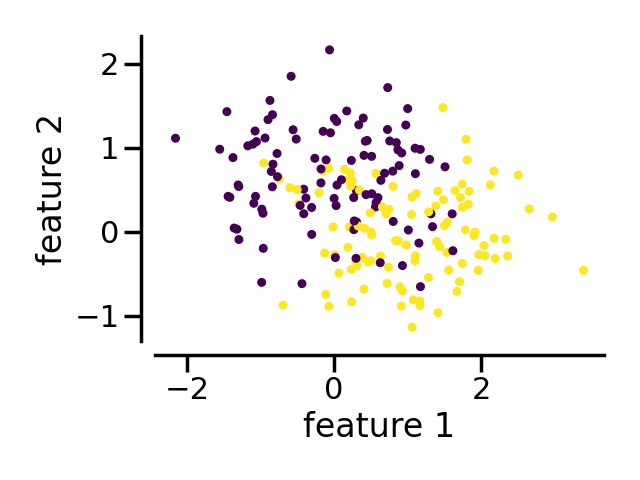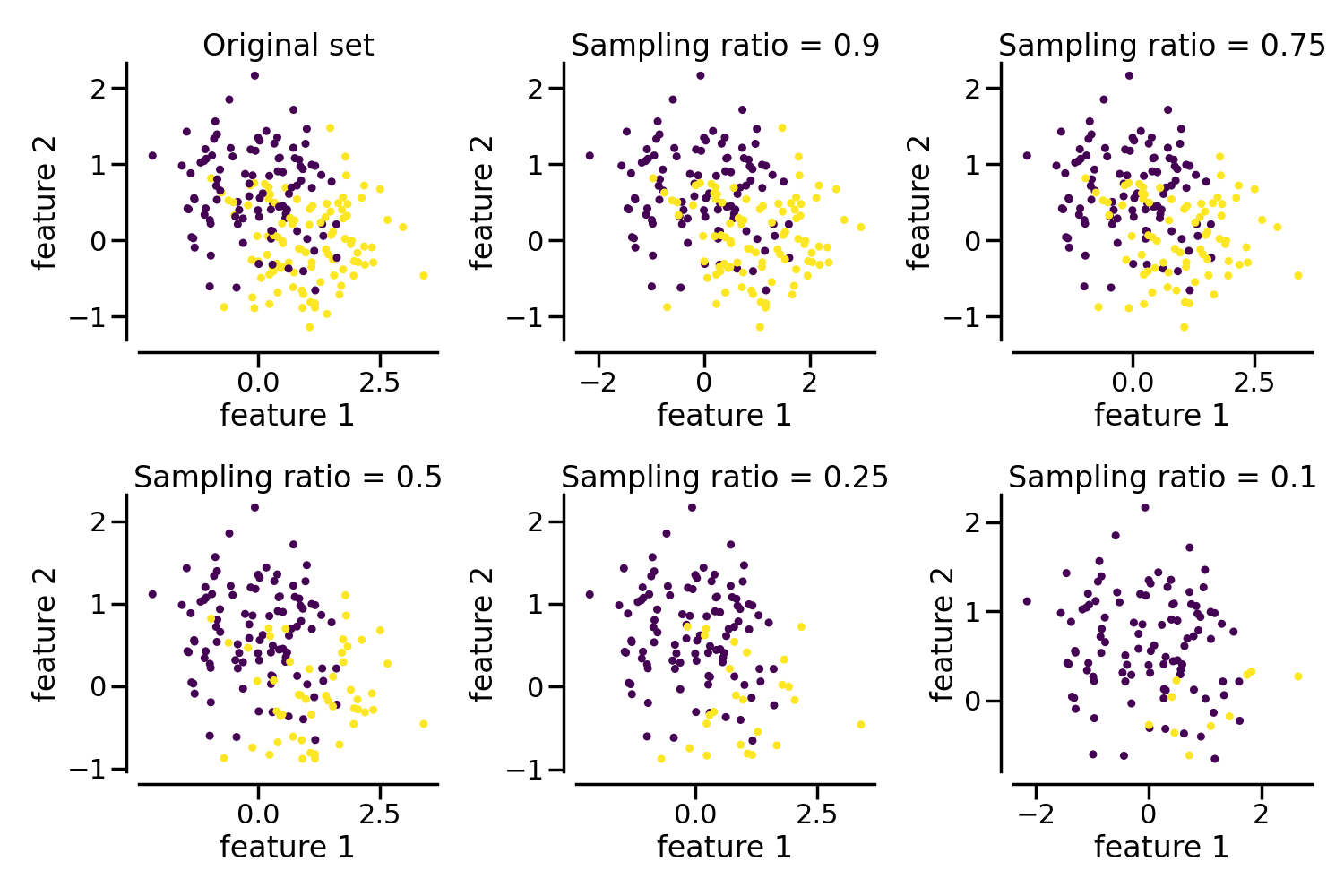# Create an imbalanced dataset#

An illustration of the `make_imbalance` function to create an imbalanced dataset from a balanced dataset. We show the ability of `make_imbalance` of dealing with Pandas DataFrame.

```# Authors: Dayvid Oliveira
#          Christos Aridas
#          Guillaume Lemaitre <g.lemaitre58@gmail.com>
```
```print(__doc__)

import seaborn as sns

sns.set_context("poster")
```

## Generate the dataset#

First, we will generate a dataset and convert it to a `DataFrame` with arbitrary column names. We will plot the original dataset.

```import matplotlib.pyplot as plt
import pandas as pd
from sklearn.datasets import make_moons

X, y = make_moons(n_samples=200, shuffle=True, noise=0.5, random_state=10)
X = pd.DataFrame(X, columns=["feature 1", "feature 2"])
ax = X.plot.scatter(
x="feature 1",
y="feature 2",
c=y,
colormap="viridis",
colorbar=False,
)
sns.despine(ax=ax, offset=10)
plt.tight_layout()
```## Make a dataset imbalanced#

Now, we will show the helpers `make_imbalance` that is useful to random select a subset of samples. It will impact the class distribution as specified by the parameters.

```from collections import Counter

def ratio_func(y, multiplier, minority_class):
target_stats = Counter(y)
return {minority_class: int(multiplier * target_stats[minority_class])}
```
```from imblearn.datasets import make_imbalance

fig, axs = plt.subplots(nrows=2, ncols=3, figsize=(15, 10))

X.plot.scatter(
x="feature 1",
y="feature 2",
c=y,
ax=axs[0, 0],
colormap="viridis",
colorbar=False,
)
axs[0, 0].set_title("Original set")
sns.despine(ax=axs[0, 0], offset=10)

multipliers = [0.9, 0.75, 0.5, 0.25, 0.1]
for ax, multiplier in zip(axs.ravel()[1:], multipliers):
X_resampled, y_resampled = make_imbalance(
X,
y,
sampling_strategy=ratio_func,
**{"multiplier": multiplier, "minority_class": 1},
)
X_resampled.plot.scatter(
x="feature 1",
y="feature 2",
c=y_resampled,
ax=ax,
colormap="viridis",
colorbar=False,
)
ax.set_title(f"Sampling ratio = {multiplier}")
sns.despine(ax=ax, offset=10)

plt.tight_layout()
plt.show()
```Total running time of the script: ( 0 minutes 1.474 seconds)

Estimated memory usage: 9 MB

Gallery generated by Sphinx-Gallery